## Indicators

•Cited by SciELO
•Access statistics

•Similars in SciELO

## Print version ISSN 0035-001X

### Rev. mex. fis. vol.65 n.4 México Jul./Aug. 2019  Epub May 06, 2020

#### https://doi.org/10.31349/revmexfis.65.333

Research

Any l-state solutions of the Schrödinger equation for q-deformed Hulthen plus generalized inverse quadratic Yukawa potential in arbitrary dimensions

aTheoretical Physics Group, Department of Physics, University of Port Harcourt, Choba, Nigeria

bDepartment of Physics, University of Calabar, Etagbor, PMB 1115, Calabar, Nigeria. e-mail: collinsokonedet@gmail.com

Abstract

The bound state approximate solution of the Schrodinger equation is obtained for the q-deformed Hulthen plus generalized inverse quadratic Yukawa potential (HPGIQYP) in D-dimensions using the Nikiforov-Uvarov (NU) method and the corresponding eigenfunctions are expressed in Jacobi polynomials. Seven special cases of the potential are discussed and the numerical energy eigenvalues are calculated for two values of the deformation parameter in different dimensions.

Keywords: Schrodinger equation; q-deformed potential; Hulthen potential (HP); generalized inverse quadratic Yukawa potential (GIQYP); Nikiforv-Uvarov (NU)

PACS: 03.65.w; 03.65.Fd; 03.65.Ge

1. Introduction

The Non Relativistic Schrödinger equation (SE) is one of the central equations in quantum physics which still attracts strong interest of both physicists and mathematicians. However, solving this equation is often very difficult, also obtaining exact analytic solutions may be found only for few cases . Many advanced mathematical methods have been used to solve it. Among the most popular methods are Nikiforov-Uvarov method (NU) [2-10], asymptotic iteration method (AIM) [11-16], supersymmetric shape invariance approach (SUSY QM) [17-22], factorization method , exact/proper quantization rule [24-26], 1/N shifted expansion method  and Modified Factorisation Method [28-29] which could help to obtain approximate solutions of these wave equations in the presence of a suitable approximation scheme.

Hulthen potential is one of the crucial molecular potentials used in different areas of Physics such as nuclear and particle, atomic and condensed matter Physics and chemical Physics to describe the interaction between two atoms . The q deformed Hulthen potential is of the form 

V(r)=-V0e-2αr1-qe-2αr (1)

 noted that there are no differences between the behavior of the modified Yukawa potential and the inversely quadratic Yukawa potential  or the Yukawa potential . Its application to diverse areas of physics has been of great interest and concern in recent times [33,34].

The generalized inverse quadratic Yukawa potential (GIQYP) is a superposition of the inverse quadratic Yukawa (IQY) and the Yukawa potential. It is asymptotic to a finite value as r → ∞ and becomes infinite at r = 0 . The Generalized inverse quadratic Yukawa potential model is of the form 

Vr=-V11+e-αrr2

Vr=-C-Be-αrr-Ae-αrr2 (2)

A=C=V1 and B=2V1

The Generalized inverse quadratic Yukawa potential reduces to a constant potential when A = B = 0.

The study of dimensions plays an important role in many areas of physics and the extension of physical problems to higher dimensional space is of great interest.  noted that the exact solutions of both the relativistic and nonrelativistic wave equation with certain physical potential in higher dimensions are remarkably important not only in physics and chemistry, but also in pure and applied mathematics.

Recently, there has been great interest in combining of two or more potentials in both the relativistic and non-relativistic regime. The essence of combining two or more physical potential models is to have a wider range of applications . For example, Hellmann , studied Schrödinger equation with a superposition of Coulomb potential and Yukawa potential, this potential is named Hellmann potential. His result is applicable in the area where both Coulomb potential and Yukawa potential respectively find applications. Bearing this in mind, we attempt to study the D-dimensional Schrödinger equation with a newly proposed potential obtained from a combination of q -deformed Hulthen potential (1) and Generalized inverse quadratic Yukawa potential (2). The proposed potential is of the form;

V(r)=-V0e-2αr1-qe-2αr-V11+e-αrr2 (3)

where V1 is the coupling strength of the potential, α is the screening parameter and V0 is strength of the potential.

The organization of the work is as follows: In the next section, review the Nikiforov-Uvarov method. In Sec. 3, this method is applied to obtain the bound state solutions. In Sec. 4, we obtain numerical results while in Sec. 5 we discuss some special cases and in Sec. 6, we give the concluding remark.

2. Review of Nikiforov-Uvarov method

The Nikiforov-Uvarov (NU) method is based on solving the hypergeometric-type second-order differential equations by means of the special orthogonal functions . The main equation which is closely associated with the method is given in the following form [40-41]

ψ(s)+τ̃(s)σ(s)ψ'(s)+σ̃(s)σ2(s)ψ(s)=0. (4)

Where σ(s) and σ(s) are polynomials at most second-degree, τ̃(s) is a first-degree polynomial and ψ(s) is a function of the hypergeometric-type.

The exact solution of Eq. (2) can be obtained by using the transformation

ψ(s)=ϕ(s)y(s) (5)

This transformation reduces Eq. (2) into a hypergeomet- ric-type equation of the form

σ(s)y(s)+τ(s)y'(s)+λy(s)=0 (6)

The function Φ(s) can be defined as the logarithm derivative

ϕ'(s)ϕ(s)=π(s)σ(s) (7)

where

π(s)=12[τ(s)-τ̃(s)] (8)

With π(s) being at most a first-degree polynomial. The second ψ(s), being yn (n) in Eq. (3), is the hypergeometric function with its polynomial solution given by Rodrigues relation

y(n)(s)=Bnρ(s)dndsn[σnρ(s)] (9)

Here, Bn is the normalization constant and ρ(s) is the weight function which must satisfy the condition

(σ(s)ρ(s))'=σ(s)τ(s) (10)

τ(s)=σ̃(s)+2π(s). (11)

It should be noted that the derivative of τ(s) with respect to s must be negative. The eigenfunctions and eigenvalues can be obtained using the definition of the following function π(s) and parameter λ, respectively:

πs=σ's-τ~s2±σ's-τ~s22-σ~s+kσs (12)

where

k=λ-π'(s) (13)

The value of k can be obtained by setting the discriminant of the square root in Eq. (9) equal to zero. As such, the new eigenvalue equation can be given as

λn=-nτ'(s)-n(n-12σ(s),n=0,1,2, (14)

3. Bound state solution with q deformed Hulthen and generalized inverse quadratic Yukawa potential in D dimension

The radial Schrödinger equation in D dimension can be written as :

d2Rnldr2-2μVr2D+2l-1D+2l-34r2+2μEnl2Rnlr=0 (15)

where μ is the reduced mass, Enl is the energy spectrum, ħ is the reduced Planck’s constant and n and l are the radial and orbital angular momentum quantum numbers respectively (or vibration-rotation quantum number in quantum chemistry). Substituting Eq. (1) into Eq. (15) gives:

d2Rnldr2-2μ2-V0e-2αr1-qe-2αr-V11+e-αrr2-D+2l-1D+2l-34r2+2μEnl2Rnlr=0 (16)

Simplifying further Eq. (16) becomes;

d2dr2-2μ2-V0e-2αr1-qe-2αr-C-Be-αrr-Ae-2αrr2-D+2l-1D+2l-34r2+2μEnl2Rnlr=0 (17)

Employing the Pekeris type approximation scheme , which is given by

1r2=4α2e-2αr1-qe-2αr2

1r=2αe-2αr1-qe-2αr (18)

Eq. (18) becomes;

d2Enlrdr2+11-qe-2αr22μEnl+C2x1-qe-2αr2+2μV0e-2αr21-qe-2αr+4μBαe-2αr21-qe-2αr+8μAα2e-4αr2-D+2l-1D+2l-34α2e-2αr4Rnl (19)

Eq. (19) can be simplified introducing the following dimensionless abbreviations

*20l-εn=μ(Enl+C)22α2δ=μV022α2χ=μB2αη=2μA2αγ=(D+2l-1)(D+2l-3)4 (20)

And using the transformation s = e-2αr so as to enable us apply the NU method as a solution of the hypergeometric type

d2Enlrdr2=4α2s2d2Enlsds2+4α2sdEnlsds (21)

d2Rnlsds2+1+qss1-qsd2Rnlsds+1s21-qs2x-s2εnq2+δq+xq-η+s2εnq+δ+x+γ-εnRnls=0 (22)

Comparing Eq. (19) and Eq. (2), we have the following parameters

*20cτ̃(s)=1-qsσ(s)=s(1-qs)σ̃(s)=-s2(εnq2+δq+χq-η)+s(2εnq2+δq+χq-η)-εn (23)

Substituting these polynomials into Eq. (9), we get π(s) to be

π(s)=-qs2±(a-k)s2+(b+k)s+c (24)

where

*20ca=q24+εnq2+δq+χq-ηb=-(2εnq+δ+χ-γ)c=εn (25)

To find the constant k the discriminant of the expression under the square root of Eq. (21) must be equal to zero. As such, we have that

k±=(χ+δ-γ)±2qεn14-ηq2+γq (26)

Substituting Eq. (26) into Eq. (24) yields

πs=-qs2±q14-ηq2+Υqs-εn (27)

From the knowledge of NU method, we choose the expression π(s) whose function τ(s) has a negative derivative. This is given by

k_=(χ+δ-γ)-2qεn14-ηq2+γq (28)

with τ(s) being obtained as

τs=1-2qs-2q14-ηq2+Υq+qεns-εn (29)

Referring to Eq. (10), we define the constant λ as

λ=-q2-q14-ηq2+Υq+qεn+x+δ-Υ-2qεn14-ηq2+γq (30)

Substituting Eq. (27) into Eq. (11) and carrying out simple algebra, where

τ's=-2q+q14-ηq2+Υq +qεns<0 (31)

and

σ(s)=-2q, (32)

we have

εn=14n+12+14-ηq2+γq2+ηq2-δq-χqn+12+14-ηq2+γq2 (33)

Substituting Eqs. (17) into Eq. (30) yields the energy eigenvalue equation of the q-deformed Hulthen potential and Generalized Inverse Quadratic Yukawa Potential in D dimension in the form

Enl=-C-2α22μn+12+14-2μA2q2+(D+2l-1)(D+2l-3)4q2+2μA2q2-μV022qα2-μB2qαn+12+14-2μA2q2+(D+2l-1)(D+2l-3)4q2 (34)

The corresponding wave functions can be evaluated by substituting π(s) and σ(s) from Eq. (27) and Eq. (23) respectively into Eq. (7) and solving the first order differential equation. This gives

ϕ(s)=sεn(1-qs)1/2+1/4-η/q2+γ/q (35)

The weight function ρ(s) from Eq. (7) can be obtained as

ρ(s)=s2εn(1-S)21/4-η/q2+γ/q (36)

From the Rodrigues relation of Eq. (6), we obtain

yn(s)Nn,lPn(2εn,21/4-η/q2+γ/q)(1-2qs) (37)

where Pn(θ,ϑ) is the Jacobi Polynomial.

Substituting Φ(s) and yn (s) from Eq. (32) and Eq. (34) respectively into Eq. (3), we obtain

φns=Nn,lSϵn1-qs12+14-ηq2+Υq×Pn2εn,214-ηq2+Υq1-2qs (38)

4. Deductions from Eq. (34)

In this section, we take some adjustments of constants in Eq. (1) and (2) to have the following cases:

Coulomb plus inverse-square potential

If we set C = 0, α → 0, q = 1 Eq. (3) reduces to the Coulomb plus Inverse-Square Potential [44,58]:

Vr=-Br+Ar2 (39)

A=C=-V1

B=2V1

Enl=-2μB222n+1+8μA2+D+2l-22 (40)

Eq. (36) is also known as the Kratzer-Feus potential, this potential was studied by Ref.  in arbitrary dimensions. If we set ħ = µ = 1, Eq. (36) fully agrees with the result reported in Eq. (28) of Ref. . Eq. (37) is also consistent with the result obtained in Eq. (15) of Ref .

If V 0 = 0, q = 1 Eq. (3) reduces to the Generalized Inverse Quadratic Yukawa potential

V(r)=-V11+e-αrr2 (41)

and the energy Eq. (34) becomes

Enl=-C-2α22μn+12+14-2μA2+(D+2l-1)(D+2l-3)42+2μA2-μB22αn+12+14-2μA2+(D+2l-1)(D+2l-3)42 (42)

Equation (42) is the Energy Eigenvalue Equation for Generalised Inverse Quadratic Yukawa potential in D dimension. When D = 3, Eq. (42) is in full agreement with the results in Eq. (27) of Refs. .

Hulthen potential

If V1 = 0, and V0 = Ze2α Eq. (3) reduces to the q deformed Hulthen potential

V(r)=Ze2αe-2αr1-qe-2αr (43)

and the energy equation Eq. (31) becomes

Enl=-2α22μn+12+14+(D+2l-1)(D+2l-3)4q2-μV022qα2n+12+14+(D+2l-1)(D+2l-3)4q2 (44)

Equation (44) is the energy equation for the q deformed Hulthen potential in D Dimensions. If we set q = 1, α → α/2, it reduces to the energy equation for the Hulthen potential in D dimension

Enl= -2α22μ2μV02α22n+1+D+2l-2-2n+1+D+2l-242 (45)

Equation (44) is identical with the energy eigenvalue equation given in Eq. (33) of Ref. . Mores so, if we set D = 3, we arrive at the energy eigenvalue equation for the Hulthen potential in 3D.

Enl= -2α22μμV02α2n+l+1-n+l+122 (46)

Equation (46) is identical to the energy eigenvalues formula given in Eq. (34) of Ref. , Eq. (35) of Ref. , Eq. (27) of Ref.  and, Eq. (31) of Ref.  and Eq. (39) of Ref. .

Kratzer potential

If α → 0 and V0 = 0, q = 1 and setting A = -V1, B = 2V1 and C = -V1, Eq. (3) reduces to

V(r)=C-Br+Ar2. (47)

Equation (31) becomes

Vr=C-Br+Ar2 (48)

Equation (48) is the Energy eigenvalue equation for the Kratzer potential in D dimensions. If D = 3 reduces to energy equation for Kratzer potential in 3D, which is very consistent with the result obtained in Eq. (28) of Ref. 

If V0 = 0, q = 0 and B = C = 0 the potential Eq. (3) reduces to the Inverse Quadratic Yukawa Potential :

V(r)=-Ae-2αrr2 (49)

Eq. (34) becomes

Enl=-2α22μn+12+14+2μA2+(D+2l-1)(D+2l-3)42-2μA2n+12+14-2μA2+(D+2l-1)(D+2l-3)42 (50)

Equation (50) is the energy equation for the Inverse Quadratic Yukawa Potential in D Dimensions. If D = 3, Eq. (50) reduces to the energy equation in 3D, which is identical to the results in Eq. (40) of Ref. , Eq. (21) of Ref.  and Eq. (50) of Ref. .

Yukawa potential

If V0 = 0, and A = C = 0 the potential Eq. (3) reduces to the Yukawa Potential

V(r)=-Be-αrr (51)

Enl=-2α22μn+12+14+(D+2l-1)(D+2l-3)42-μB2αn+12+14+(D+2l-1)(D+2l-3)42 (52)

Equation (52) is the energy eigenvalue equation for the Yukawa potential in D dimensions. If D = 3, Eq. (52) becomes identical with Eqs. (50) and (17) reported in Ref.  and , respectively.

q Deformed Hulthen potential plus inversely quadratic Yukawa potential

If B = C = 0 the potential Eq. (3) reduces to the q Deformed Hulthen Potential plus Inversely Quadratic Yukawa Potential

V(r)=-V0e-2αr1-qe-2αr-Ae-2αrr2 (53)

Enl=-2α22μn+12+14-2μA2q2+(D+2l-1)(D+2l-3)4q2+2μA2q2-μV022qα2n+12+14-2μA2q2+(D+2l-1)(D+2l-3)4q2 (54)

Equation (51) is the energy equation for qDHPIQYP in D Dimensions. If D = 3, Eq. (51) reduces to the energy equation for qDHPIQYP in 3D. Hence, this is in agreement with the result reported in Eq. (21) of Ref. .

Wood saxon

If V1 =0, q → 0, the potential Eq. (3) reduces to the Wood-Saxon Potential 

V(r)=-V0e-2αr1+e-2αr (55)

Enl=-2α22μn+12+14-(D+2l-1)(D+2l-3)42+μV022α2n+12+14-(D+2l-1)(D+2l-3)42 (56)

Equation (56) is the energy equation for Woood saxon potential in D Dimensions. If D = 3, Eq. (56) reduces to energy equation for Wood Saxon potential in 3D which is in agreement with Eq. (33) of Ref. [51,52].

Coulomb potential

If V0 = 0, A = C = 0, α → 0the potential Eq. (3) reduces to the Coulomb Potential 

V(r)=-Br (57)

Enl=-μB222n+12+14+(D+2l-1)(D+2l-3)42 (58)

Equation (58) is the energy equation for Coulomb potential in D Dimensions. This result is consistent with the result obtained in Eq. (7.14) of Ref. . Also, comparing our work with the result gotten in Eq. (32) of Ref. , it is worthy to note here that the authors in Ref.  failed to set the screening parameter (i.e. δ in Eq. (32) of Ref. ) equal to zero. If that is done, then there would be a clear consistency in the energy eigenvalue equation gotten in our Eq. (58) and Eq. (32) of Ref. . More so, when D = 3, Eq. (58) reduces to the energy equation for Coulomb potential in 3D. This result is in agreement with the result obtained in Eq. (101) of Ref. .

5. Discussion

In Table I, we present the numerical results for q-deformed HPGIQYP in natural units for undisturbed system q = 1 and low potential strength, V0 = 0.05 eV and coupling strength eV. It is observed that the energy decreases as the orbital angular momentum increases for any given value of the principal quantum number n in all dimensions. Physically, a particle in this potential is highly bound and becomes more attractive as increases. Interestingly, the above observation is the same with and without the presence of the deformation parameter in the system as shown in Table II for which q =2.

Table I The bound state energy levels (in units of fm-1) of the q deformed HPGIQYP for various values of n, l and for ħ = μ = 1, V 1 = 0:05, V 0 = 0:06, α = 0:1 and q = 1.

 D l E0 E1 E2 E3 E4 E5 1 0 -0.111532657 -0.050160469 -0.061805001 -0.091588170 -0.133612341 -0.186495563 1 -0.111532657 -0.050160469 -0.061805001 -0.091588170 -0.133612341 -0.186495563 2 -0.050001470 -0.063637479 -0.094491464 -0.137403514 -0.191119393 -0.255212929 3 -0.063963864 -0.095000000 -0.138064566 -0.191923889 -0.256157355 -0.330589834 2 0 - - - - - - 1 -0.057730423 -0.053663600 -0.076861376 -0.113796613 -0.162002377 -0.220756343 2 -0.054040856 -0.077671011 -0.114918947 -0.163406670 -0.222431895 -0.291727413 3 -0.077934315 -0.115282910 -0.163861568 -0.222974330 -0.292355951 -0.371887520 3 0 -0.111532657 -0.050160469 -0.061805001 -0.091588170 -0.133612341 -0.186495563 1 -0.050001470 -0.063637479 -0.094491464 -0.137403514 -0.191119393 -0.255212929 2 -0.063963864 -0.095000000 -0.138064566 -0.191923889 -0.256157355 -0.330589834 3 -0.095215754 -0.138344769 -0.192264742 -0.256557384 -0.331048371 -0.415654558 4 0 -0.057730423 -0.053663600 -0.076861376 -0.113796613 -0.162002377 -0.220756343 1 -0.054040856 -0.077671011 -0.114918947 -0.163406670 -0.222431895 -0.291727413 2 -0.077934315 -0.115282910 -0.163861568 -0.222974330 -0.292355951 -0.371887520 3 -0.115463942 -0.164087740 -0.223243964 -0.292668339 -0.372242321 -0.461906307 5 0 -0.050001470 -0.063637479 -0.094491464 -0.137403514 -0.191119393 -0.255212929 1 -0.063963864 -0.095000000 -0.138064566 -0.191923889 -0.256157355 -0.330589834 2 -0.095215754 -0.138344769 -0.192264742 -0.256557384 -0.331048371 -0.415654558 3 -0.138499958 -0.192453483 -0.256778864 -0.331302223 -0.415940595 -0.510650327

Table II The bound state energy levels (in units of fm-1) of the q deformed HPGIQYP for various values of n, l and for ħ = μ = 1,

 D l E0 E1 E2 E3 E4 E5 1 0 -0.055540550 -0.054743460 -0.076688104 -0.110461693 -0.154758568 -0.209260208 1 -0.055540550 -0.054743460 -0.076688104 -0.110461693 -0.154758568 -0.209260208 2 -0.050713075 -0.067097009 -0.096794134 -0.137281881 -0.188051277 -0.248945654 3 -0.060304344 -0.086357884 -0.123577555 -0.171174400 -0.228929320 -0.296761967 2 0 -0.062855099 -0.052655813 -0.072364751 -0.104407142 -0.147071045 -0.199969056 1 -0.050377886 -0.059888551 -0.085683732 -0.122678251 -0.170057863 -0.227598190 2 -0.054379255 -0.075979470 -0.109478988 -0.153516061 -0.207762252 -0.272106443 3 -0.068012053 -0.098139551 -0.139023045 -0.190178724 -0.251455966 -0.322795574 3 0 -0.055540550 -0.054743460 -0.076688104 -0.110461693 -0.154758568 -0.209260208 1 -0.050713075 -0.067097009 -0.096794134 -0.137281881 -0.188051277 -0.248945654 2 -0.060304344 -0.086357884 -0.123577555 -0.171174400 -0.228929320 -0.296761967 3 -0.077274238 -0.111271926 -0.155781564 -0.210492483 -0.275298367 -0.350154842 4 0 -0.050377886 -0.059888551 -0.085683732 -0.122678251 -0.170057863 -0.227598190 1 -0.054379255 -0.075979470 -0.109478988 -0.153516061 -0.207762252 -0.272106443 2 -0.068012053 -0.098139551 -0.139023045 -0.190178724 -0.251455966 -0.322795574 3 -0.087972214 -0.125723307 -0.173833646 -0.232096027 -0.300433652 -0.378812734 5 0 -0.050713075 -0.067097009 -0.096794134 -0.137281881 -0.188051277 -0.248945654 1 -0.060304344 -0.086357884 -0.123577555 -0.171174400 -0.228929320 -0.296761967 2 -0.077274238 -0.111271926 -0.155781564 -0.210492483 -0.275298367 -0.350154842 3 -0.100039710 -0.141473606 -0.193167160 -0.254977708 -0.326848638 -0.408753857

Figure 1-5 show the variations of potential versus r for different values of q, considering three potentials and four orbital angular momentums. In Figs. 6 and 7, we show the 3D variations of the energy with q for s-wave and p-state for different n. We repeat the same for the screening parameter α in Fig. 8 and 9. The energy increases when the potential strength V 0 increases, but behaves the other way roundfor coupling strength V1 as shown in Fig. 10-13. Finally, Figs. 14-17 shows the variation of energy level for various dimension D for the s-wave and p-state. The energy decreases when the dimension D increases in both cases and peaks at D = 2.Figure 1 The variation of the q deformed Hulthen potential plus Generalized inverse quadratic Yukawa potential for various values of the deformation parameter (q+) as a function r. We choose V1 = 0:5, V0 = 0:6 and α = 0:1.Figure 2 The variation of the q deformed Hulthen potential plus Generalized inverse quadratic Yukawa potential for various values of the deformation parameter (q-) as a function r. We choose V1 = 0:5, V0 = 0:6 and α = 0:1.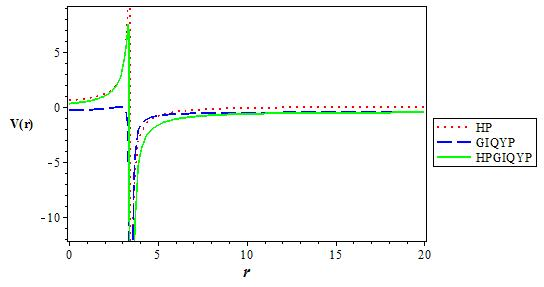Figure 3 The variation of the q deformed Hulthen potential, Generalized inverse quadratic Yukawa potential and q deformed Hulthen potential plus Generalized inverse quadratic Yukawa potential as a function r. We choose V1 = 0:5, V0 = 0:6, q = 2 and α = 0:1.Figure 4 The variation of the effective q deformed Hulthen potential plus Generalized inverse quadratic Yukawa potential for various values of l as a function r. We choose V1 = 0:5, V0 = 0:6, q = 2 and α = 0:1 in 3DFigure 5 The variation of the effective q deformed Hulthen potential plus Generalized inverse quadratic Yukawa potential for various values of l as a function r. We choose V1 = 0:5, V0 = 0:6, q = -2 and α = 0:1 in 3DFigure 6 The variation of the s state (l = 0) energy level for various n as a function of the deformation parameter. We choose V1 = 0:05, V0 = 0:06, and α = 0:1 in 3D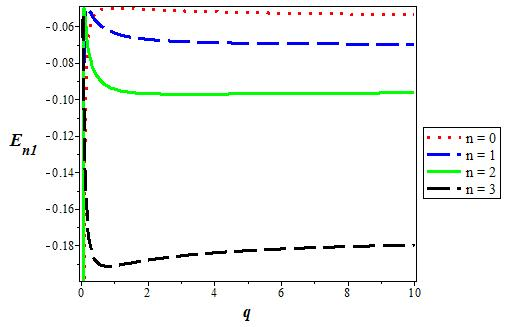Figure 7 The variation of the p state (l = 1) energy level for various n as a function of the deformation parameter. We choose V1 = 0:05, V0 = 0:06, and α = 0:1 in 3D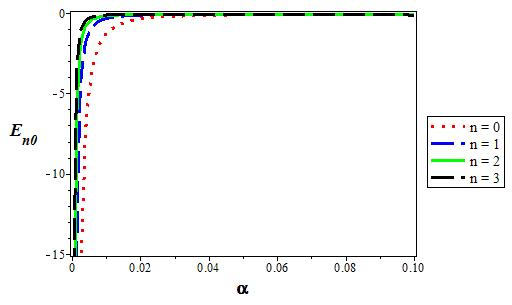Figure 8 The variation of the s state (l = 0) energy level for various n as a function of the screening parameter (α). We choose V1 = 0:05, V0 = 0:06, and q = 2 in 3D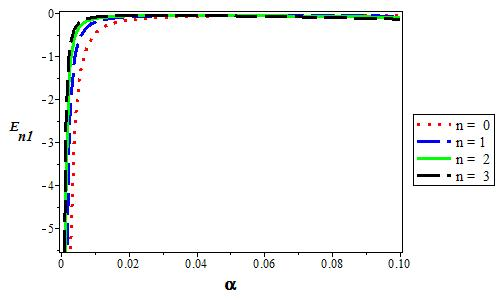Figure 9 The variation of the p state (l = 1) energy level for various n as a function of the screening parameter (α). We choose V1 = 0:05, V0 = 0:06, and q = 2 in 3D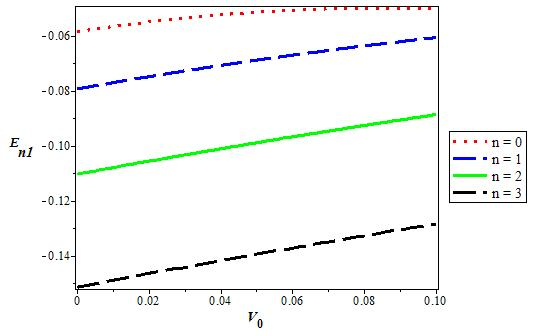Figure 10 The variation of the p state (l = 1) energy level for various n as a function of the coupling strength V0. We choose V1 = 0:05, α = 0:1, and q = 2 in 3D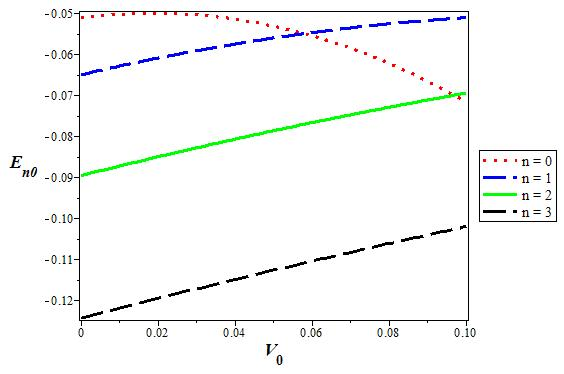Figure 11 The variation of the s state (l = 0) energy level for various n as a function of the coupling strength V0. We choose V1 = 0:05, α = 0:1, and q = 2 in 3D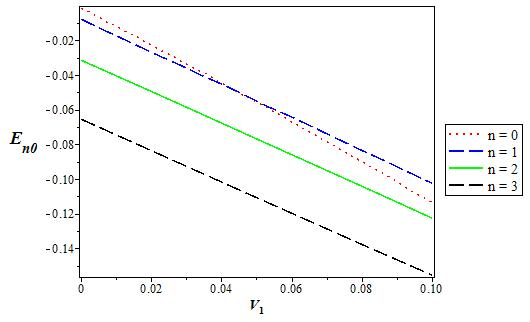Figure 12 The variation of the s state (l = 0) energy level for various n as a function of the coupling strength V 1. We choose V0 = 0:06, α = 0:1, and q = 2 in 3D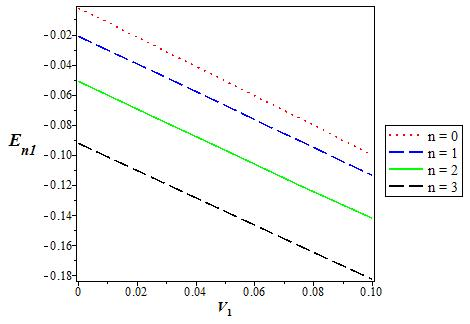Figure 13 The variation of the p state (l = 1) energy level for various n as a function of the coupling strength V1. We choose V0 = 0:06, α = 0:1, and q = 2 in 3D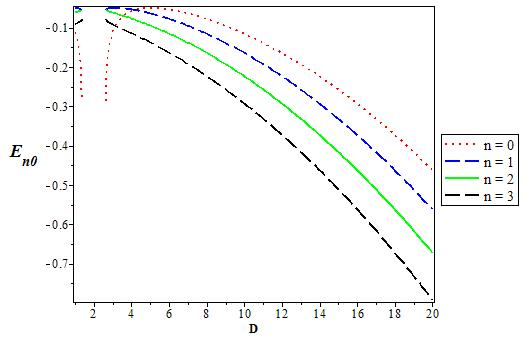Figure 14 The variation of the p state (l = 0) energy level for various D as a function of the coupling strength V1. We chooseV0 = 0:06, V1 = 0:05, α = 0:1, and q = 1.Figure 15 The variation of the p state (l = 1) energy level for various D as a function of the coupling strength V1. We choose V0 = 0:06, V1 = 0:05, α = 0:1, and q = 1.Figure 16 The variation of the s state (l = 0) energy level for various D as a function of the coupling strength V1. We choose V0 = 0:06, V1 = 0:05, α = 0:1, and q = 2.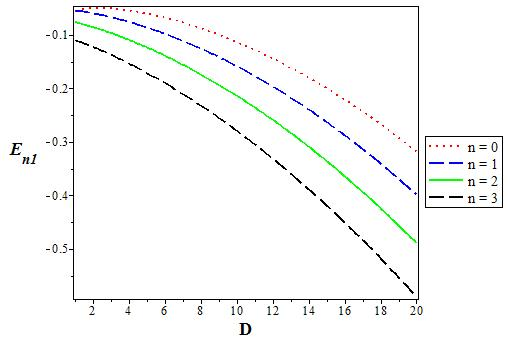Figure 17 The variation of the p state (l = 1) energy level for various D as a function of the coupling strength V1. We choose V0 = 0:06, V1 = 0:05, α = 0:1, and q = 2.

6. Conclusion

In this work, we have studied the bound state solutions of the Schrodinger equation with q deformed Hulthen plus generalized inverse quadratic Yukawa potential using NU method. By making appropriate approximation to deal with the centrifugal term, we obtain the energy eigenvalues and the corresponding eigenfunctions and also discussed some special cases of the potential. We have calculated numerical energy eigenvalues and presented plots for various values of the potential parameters and found that the energy decreases as dimension increases. The results are in excellent agreement with literature.

Acknowledgments

We are grateful to Dr. A. N. Ikot for communicating some of his research materials to us.

References

1. M. Chabab, A. El Batoul, and M. Oulne, Zeitschrift fur Naturforschung A, (2015). Source: arXiv [ Links ]

2. A. F. Nikiforov and V. B. Uvarov, Special Functions of Mathematical Physics (Birkhauser, Basel, 1988). [ Links ]

3. C.O. Edet, U.S. Okorie, A.T. Ngiangia and A.N. Ikot, Indian J Phys (2019) https://doi.org/10.1007/s12648-019-01477-9 [ Links ]

4. C.O. Edet, K.O. Okorie, H. Louis and N.A. Nzeata-Ibe. Indian J Phys (2019). https://doi.org/10.1007/s12648-019-01467-x [ Links ]

5. K.J. Oyewumi, O.J. Oluwadare, K.D. Sen, O.A. Babalola, J. Math. Chem. 51 (2013) 976-991 [ Links ]

6. B.J. Falaye, K.J. Oyewumi, M. Abbas, Chin. Phys. B. 22 (2013) 110301 [ Links ]

7. M. Hamzavi, S.M. Ikhdair, Few-Body Syst. 54 (2013) 1753-1752 [ Links ]

8. M. Eshghi, H. Mehraban, J. Scien Research 3 (2011) 239-247 [ Links ]

9. C.A. Onate, A.N. Ikot, M.C. Onyeaju, M.E. Udoh,. Karbala Int. J. Mod. Science. 3 (2017) 1-7 [ Links ]

10. C.A. Onate, M.C. Onyeaju, Sri. J. Phys. 17 (2016) 1-17 [ Links ]

11. B.J. Falaye, K.J. Oyewumi, T.T. Ibrahim, M.A. Punyasena, C.A. Onate, Can. J. Phys. 91 (2013) 98-104 [ Links ]

12. K.J. Oyewumi, B.J. Falaye, C.A. Onate, O.J. Oluwadare, W.A. Yahya, Mol. Phys. 112 (2014) 127-141 [ Links ]

13. O. Bayrak, I. Boztosun, Phys. Scr. 76 (2007) 92-96 [ Links ]

14. E. Ateser, H. Ciftci, M. Uǧurlu, Chin. J. Phys. 45 (2007) 346-351 [ Links ]

15. O. Bayrak, I. Boztosun, H. Ciftci, Int. J. Quant. Chem. 107 (2007) 540-544 [ Links ]

16. M. Aygun, O. Bayrak, I. Boztosun, J. Phys. B: Mol. Opt. Phys. 40 (2007) 537-544 [ Links ]

17. H. Hassanabadi, S. Zarrinkamar, B.H. Yazarloo, Chin. J. Phys. 50 (2012) 788-794 [ Links ]

18. A.N. Ikot, Hassanabadi, E. Maghsoodi, S. Zarrinkamar, Commun. Theor. Phys. 61 (2014) 436 [ Links ]

19. C.N. Isonguyo, I.B. Okon, A.N. Ikot, H. Hassanabadi, Bull. Korean Chem. Soc. 35 (2014) 3443 [ Links ]

20. A. N. Ikot, H.P. Obong, H. Hassanabadi, Few-Body Syst. 56 (2015) 185 [ Links ]

21. C.A. Onate and J.O. Ojonubah. Int. J. Mod. Phys. E 24 (2015) 1550020-1 [ Links ]

22. A.N. Ikot, S. Zarreinkamar, S. Zare, H. Hassanabadi, Chin. J. Phys. 54 (2016) 968-977 [ Links ]

23. S.H. Dong. Factorization Method in Quantum Mechanics. (Springer, 2007). [ Links ]

24. S.H. Dong, A. Gonzalez-Cisneros, Ann. Phys. 323 (2008) 1136-1149. [ Links ]

25. X.Y. Gu, S.H. Dong, Z.Q. Ma, J. Phys. A: Math. Theor. 42 (2009) 035303 [ Links ]

26. S.M. Ikhdair, J. Abu-Hasna, Phys. Scr. 83 (2011) 025002 [ Links ]

27. R.H. Hammed, J. Bosrah Researches. 38 (2012) 51-59 [ Links ]

28. Uduakobong S. Okorie Akpan N. Ikot, Michael C. Onyeaju and Ephraim O. Chukwuocha, J. of Mol. Mod. 24 (2018) 289. https://doi.org/10.1007/s00894-018-3811-8 [ Links ]

29. U. S. Okorie and E. E. Ibekwe, A. N. Ikot, M. C. Onyeaju and E. O. Chukwuocha, Journal of the Korean Physical Society, 73 (2018) 1211-1218. [ Links ]

30. C. A. Onate, SOP Trans on Theoretical Physics, 1 (2014) 2. [ Links ]

31. M. Hamzavi, S. M. Ikhdair and B. I. Ita, Phys. Scr. 85 (2012) 045009. [ Links ]

32. S. M Ikhdair, Cent. Eur. J. Phys. 10 (2012) 361. [ Links ]

34. H. Taseli, Int. J. Quant. Chem. 63 (1997) 949. [ Links ]

35. Ituen B. Okon, Oyebola Popoola, and Cecilia N. Isonguyo, Adv. in High Energy Physics, (2017). https://doi.org/10.1155/2017/9671816 [ Links ]

36. Oluwatimilehin Oluwadare, Kayode Oyewumi, Chin. Phys. Lett. 34 (2017) 110301. [ Links ]

37. S.H. Dong Wave Equation in Higher Dimensions (Berlin: Springer 2011). [ Links ]

38. C.A. Onate, O. Ebomwonyi, K.O. Dopamu, J.O. Okoro, M.O. Oluwayemi, Chinese Journal of Physics (2018). doi: 10.1016/j.cjph.2018.03.013 [ Links ]

39. H. Hellmann, J. Chem. Phys. 3 (1935) 61. [ Links ]

40. G. Sezgo, Orthogonal Polynomials. American Mathematical Society, New York (1939). [ Links ]

41. C. Birkdemir, Application of the Nikiforov-Uvarov Method in Quantum Mechanics, Chapter 11 in Theoretical Concept of Quantum Mechanics, Ed. M. R. Pahlavani (2012). [ Links ]

42. Jie Gao, Min-Cang Zhang, Chin. Phys. Lett. 33 (2016) 010303. [ Links ]

43. C. I. Pekeris, Phys. Rev. 45 (1934) 98. [ Links ]

44. Shi-Hai Dong and Guo-Hua Sun, Physica Scripta 70 (2004) 94-97. [ Links ]

45. C. A. Onate, Afr. Rev. of Phys. 8 (2013) 325. [ Links ]

46. C.A. Onate and J.O. Ojonubah, J Theor Appl Phys. DOI 10.1007/s40094-015-0196-2 [ Links ]

47. Sameer M. Ikhdair, Majid Hamzavi, Few-Body Syst 53 (2012) 487-498. DOI 10.1007/s00601-012-0475-2 [ Links ]

48. Ituen B. Okon, Oyebola Popoola, Cecilia N. Isonguyo and Akaninyene D. Antia, Physical Science International Journal 19 (2018) 1-27. [ Links ]

49. C.N. Isonguyo, K.J. Oyewumi and O.S. Oyun, Int J Quantum Chem. (2018); e25620. https://doi.org/10.1002/qua.25620 [ Links ]

50. A. Berkdemir, C. Berkdemir and R. Sever, Modern Phys. Lett. A 21 (2006) 2087. [ Links ]

51. Akaninyene D. Antia and Ita O. Akpan, Adv. in Physics Theories and Applications 46 (2015). https://www.iiste.org/. [ Links ]

52. O.A. Awoga, A.N. Ikot and J.B. Emah, Rev. Mex. Fis. 59 (2013) 229-235. [ Links ]

53. D. Agboola, Phys. Scr. 80 (2009) 065304. doi: 10.1088/0031-8949/80/06/065304 [ Links ]

54. O. Bayrak, G. Kocak, I. Boztosun, J. Phys. A: Math. Gen. 39 (2006) 11521. [ Links ]

55. C.-S. Jia, J.-Y. Liu, P.-Q. Wang, Phys. Lett. 372 (2008) 4779. [ Links ]

56. S.M. Ikhdair, R. Sever, J. Math. Chem. 42 (2007) 461. [ Links ]

57. S.M. Ikhdair, Eur. Phys. J. A 39 (2009) 307-314. [ Links ]

58. K. J. Oyewummi, Foundations of Physics Letters (2005) DOI: 10.1007/s10702-005-2481-9 [ Links ]

Received: December 18, 2018; Accepted: January 25, 2019This is an open-access article distributed under the terms of the Creative Commons Attribution License